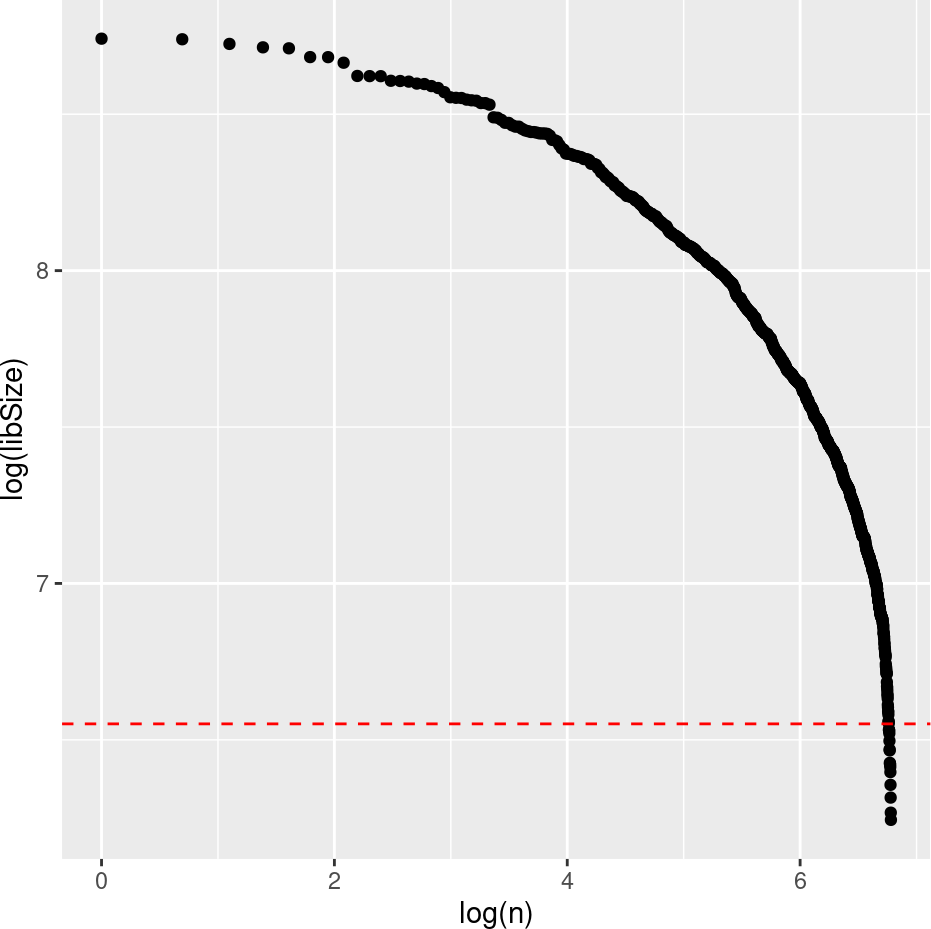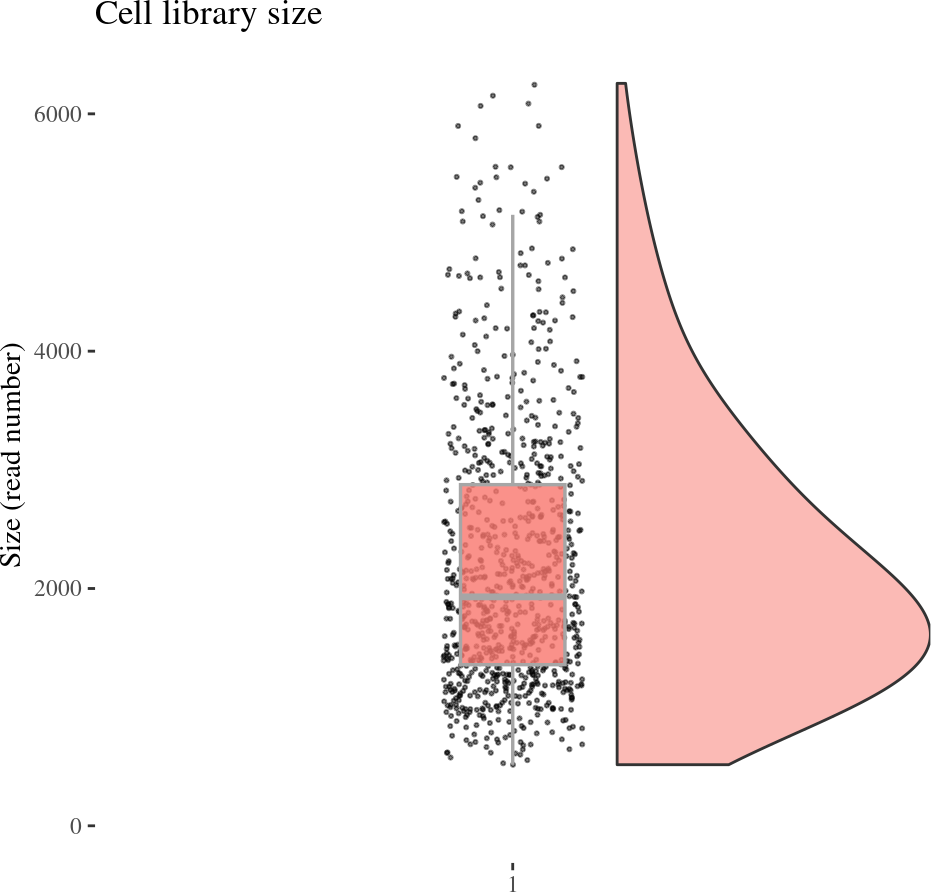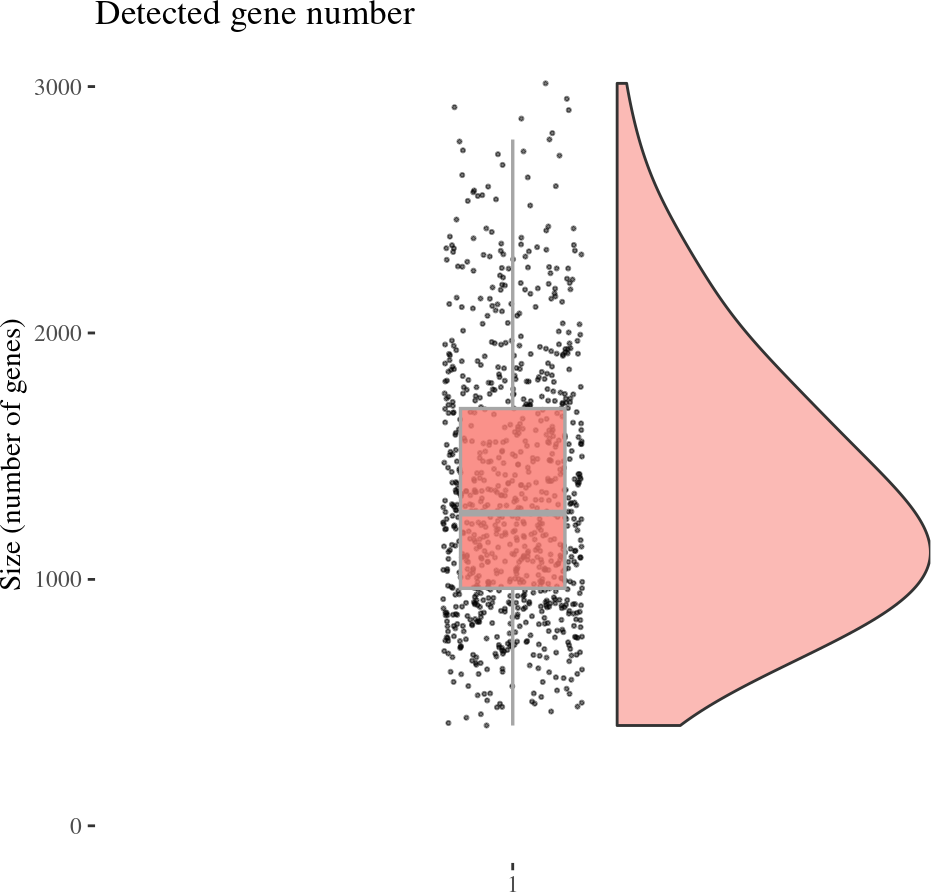# Contents

``````library(COTAN)
library(zeallot)
library(data.table)
library(factoextra)
library(Rtsne)
library(qpdf)
library(GEOquery)

options(parallelly.fork.enable = TRUE)``````

## 0.1 Introduction

This tutorial contains the same functionalities as the first release of the COTAN tutorial but done using the new and updated functions.

## 0.2 Get the data-set

Download the data-set for `"mouse cortex E17.5"`.

``````dataDir <- tempdir()

GEO <- "GSM2861514"
fName <- "GSM2861514_E175_Only_Cortical_Cells_DGE.txt.gz"

if (!file.exists(dataSetFile)) {
getGEOSuppFiles(GEO, makeDirectory = TRUE,
baseDir = dataDir, fetch_files = TRUE,
filter_regex = fName)
sample.dataset <- read.csv(dataSetFile, sep = "\t", row.names = 1L)
}``````

Define a directory where the output will be stored.

``````outDir <- tempdir()

# Log-level 2 was chosen to showcase better how the package works
# In normal usage a level of 0 or 1 is more appropriate
setLoggingLevel(2L)
#> Setting new log level to 2

# This file will contain all the logs produced by the package
# as if at the highest logging level
setLoggingFile(file.path(outDir, "vignette_v2.log"))
#> Setting log file to be: /tmp/RtmpSMV3wE/vignette_v2.log``````

# 1 Analytical pipeline

Initialize the `COTAN` object with the row count table and the metadata for the experiment.

``````cond <- "mouse_cortex_E17.5"
#cond <- "test"

#obj = COTAN(raw = sampled.dataset)
obj <- COTAN(raw = sample.dataset)
GEO = GEO,
sequencingMethod = "Drop_seq",
sampleCondition = cond)
#> Initializing `COTAN` meta-data

logLevel = 1L)
#> Condition mouse_cortex_E17.5``````

Before we proceed to the analysis, we need to clean the data. The analysis will use a matrix of raw UMI counts as the input. To obtain this matrix, we have to remove any potential cell doublets or multiplets, as well as any low quality or dying cells.

## 1.1 Data cleaning

We can check the library size (UMI number) with an empirical cumulative distribution function

``ECDPlot(obj, yCut = 700L)````````cellSizePlot(obj)
#> Warning: Removed 1 rows containing missing values (`geom_point()`).````````genesSizePlot(obj)````````mit <- mitochondrialPercentagePlot(obj, genePrefix = "^Mt")
mit[["plot"]]``````